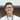# Largest number from two array

March 28, 2021

This may or may not be the optimal solution, DO NOT blindly memorize my solutions

From Cassidy’s weekly newsletter.

Target audience: people comfortable in js (please feedback!)

# This week’s question:

You’re given two integer arrays (n and m), and an integer k. Using the digits from n and m, return the largest number you can of length k.

## Example:

n = [3,4,6,5]
m = [9,0,2,5,8,3]
k = 5
$maxNum(n, m, k)$ 98655

When I first read this question, the most obvious solution was to combine the arrays then sort them, the first k numbers will be the answer. We can chain some functional functions together like so:

const maxNum = (n, m, k) =>
[...n, ...m]
.sort((a, b) => b - a)
.filter((_, i) => i < k)
.join("");

What this does is to spread n and m (O(n+m)) followed by sorting them by descending order, O(n+m log n+m) and then filtering (O(n+m)) and joining (O(k)) this gives us a complexity of O(n+m log n+m). There are many ways to implement this same logic more efficiently, but the bottle neck is the sorting.

My next idea came to me by identifying that I only needed the biggest K values, and sorting wasn’t necessary. This means that we can make use of some data structure which has a better amortised cost, such as a heap. Here’s my array implementation of a heap:

function MaxHeap() {
this.heap = [];
function getChildrenIndex(curr) {
return [2 * curr + 1, 2 * curr + 2];
}
function getParentIndex(curr) {
return curr % 2 === 0 ? (curr - 2) / 2 : (curr - 1) / 2;
}

return {
heap: this.heap,
insert: function (value) {
let { heap } = this;
heap.push(value);
let currIndex = heap.length - 1;
let parentIndex = getParentIndex(currIndex);
while (heap[currIndex] > heap[parentIndex]) {
const tmp = heap[parentIndex];
heap[parentIndex] = heap[currIndex];
heap[currIndex] = tmp;
currIndex = parentIndex;
parentIndex = getParentIndex(currIndex);
}
},
remove: function () {
let { heap } = this;
const lastItem = heap.pop();
let currIndex = 0;
let [l, r] = getChildrenIndex(currIndex);
const returnVal = heap;
heap = lastItem;
while (heap[currIndex] < heap[l] || heap[currIndex] < heap[r]) {
const tmp = heap[currIndex];
if (heap[r] > heap[l]) {
heap[currIndex] = heap[r];
heap[r] = tmp;
currIndex = r;
} else {
heap[currIndex] = heap[l];
heap[l] = tmp;
currIndex = l;
}
[l, r] = getChildrenIndex(currIndex);
}
return returnVal;
}
};
}

and then the driver function will just iteratively add n and m into the heap, with an average O(1) of insertion (O(n+m)) then removing k items (O(k)) and this gives us an average case of O(n+m) but worst case no better than the previous solution.

const maxNum = (n, m, k) => {
let h = new MaxHeap();
n.forEach((item) => h.insert(item));
m.forEach((item) => h.insert(item));
return Array(k)
.fill(0)
.map((_) => h.remove())
.join("");
};

Finally my thought went to the problem itself. Since the input are digits, we know that there can be at most 10 different input values, this allows us to do some non-comparison sorting, but we didn’t need anything as complex as radix sort, when just counting the digits would do. This solution basically counts all the digits (O(n+m)) and then goes through the count (O(10) = O(1)) and then reassembles the string. Giving us O(n+m) time complexity and a virtually constant space.

const linearMaxNum = (n, m, k) => {
const count = {};
let res = "";
let all = [...n, ...m];
all.forEach((item) => {
if (!count[item]) {
count[item] = 0;
}
count[item]++;
});
for (let i = 9; i >= 0; i--) {
res += new Array(count[i]).fill(i).join("");
}
return res.substr(0, k);
};

This question was quite interesting as I kept finding different ways to solve it, and each of them tested different part of my CS knowledge, and also some good practice in implementing a binary heap from scratch! I don’t think I can get anything better than linear, but do @ me on twitter if you find any better solutions!Ten Zhi Yang is a dev in Singapore. Disagree with me? @ me on twitter. Like my post? @ me on twitter too!